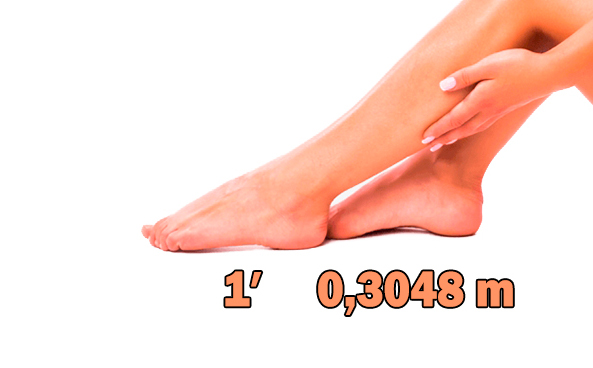Foot (′) was a very diverse unit of measure for length until 1958. In almost every country, this value was different, but then the English-speaking countries adopted the international foot as 0.3048 meters. You don’t need to memorize this, because we have already created a convenient and fast calculator for converting feet to meters. One click and you already have the desired value. Find out the height of your interlocutor in meters!

1. Originally, the foot was called the size of the foot, so in each country the length of the foot was different.
2. The designation for a foot is ′. For example, 6.5′ means six and a half feet.
3.  Foot is a non-SI unit of measure for length, but it is widely used in Europe and America.
4. Human height is often measured in feet. Now you can calculate the height of the overseas handsome in meters.

## ft to m pre-made calculations

• 5′ = 1.52 m
• 5.5’= 1.68 m
• 5.7′ = 1.74 m
• 6′ = 1.83 m
• 6.2′ = 1.89 m
• 6.5′ = 1.98 mA pictorial representation of an approximate length of one foot